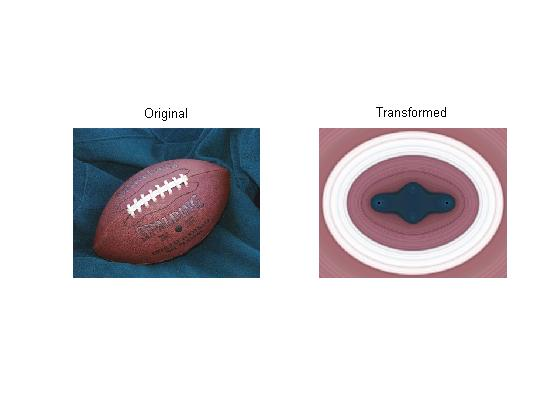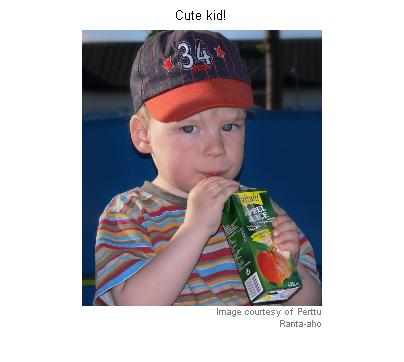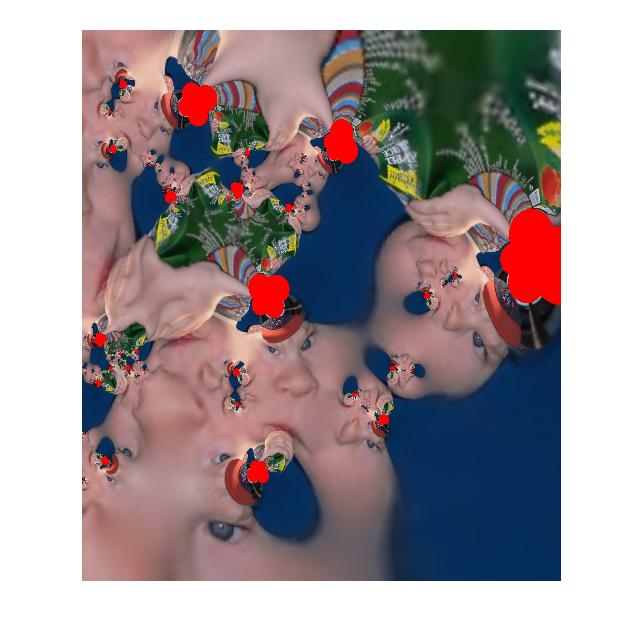Steve on Image Processing with MATLABImage processing concepts, algorithms, and MATLAB

Thanks to Kimo Johnson and Perttu Ranta-aho for responding to my custom spatial transformation challenge.

Kimo's submission

Kimo's custom transform turns the football image inside out!

sx = 1.47;
sy = 1.6;
c2 = 0.14;
c3 = 0.07;
p1 = 0.25;
p2 = 0.125;

f = @(x) complex(x(:,1)/sx,x(:,2)/sy);
g = @(z) c2*log(abs(z + p1)) + c2*log(abs(z - p1)) + ...
c3*log(abs(z + p2*i)) + c3*log(abs(z - p2*i));
h = @(w) [real(w), imag(w)];
q = @(x, unused) h(g(f(x)));

tform = maketform('custom', 2, 2, [], q, []);
trans = imtransform(im, tform, 'UData', [-1 1], 'VData', [-1 1], ...
'XData', [-1 1], 'YData', [-1 1]);

subplot(1,2,1)
imshow(im)
title('Original')

subplot(1,2,2)
imshow(trans)
title('Transformed')Perttu's submission

I really don't know how to describe this one.

Notice how Perttu used the 'FillValues' option of imtransform to get the red pixels in the output.

I'm going to shrink the image a bit so it's not too big for the blog page.

I = imresize(I, 0.5);
clf
imshow(I)
title('Cute kid!')
imcredit('Image courtesy of Perttu Ranta-aho')  % MATLAB Central File Exchangef = @(x) complex(x(:,1),x(:,2));
g = @(z) ((z-1).*(z+1).*(z+.1-2*i).*(z+1+2*i)) ./ ...
(power(z+1+i,4)+power(z-1-i,4));
h = @(w) [real(w) imag(w)];
q = @(x,unused) h(g(g(g(g(f(x))))));
tform = maketform('custom', 2, 2, [], q, []);
J=imtransform(I, tform, 'UData', [-1.5 1.5], 'VData', [-1.5 1.5], ...
'XData', [0 6], 'YData', [-4 2], 'FillValues', [255 0 0]');
imshow(J)Wow!

Published with MATLAB® 7.2

|

댓글

댓글을 남기려면 링크 를 클릭하여 MathWorks 계정에 로그인하거나 계정을 새로 만드십시오.